Noise
Lab: Probabilistic Power Spectral Densities

Seismo-Live: http://seismo-live.org

##### Authors:¶

In :
%matplotlib inline
import matplotlib.pyplot as plt
plt.style.use("bmh")
plt.rcParams['figure.figsize'] = 10, 6

• read waveform data from file data/GR.FUR..BHN.D.2015.361 (station FUR, LMU geophysical observatory in Fürstenfeldbruck)
• read corresponding station metadata from file data/station_FUR.stationxml
• print info on both waveforms and station metadata
In :
from obspy import read, read_inventory

print(st)
print(inv)
inv.plot(projection="ortho");

1 Trace(s) in Stream:
GR.FUR..BHN | 2015-12-27T00:00:09.769999Z - 2015-12-28T00:00:11.669999Z | 20.0 Hz, 1728039 samples
Inventory created at 2016-05-03T10:14:19.000000Z
Created by: JANE WEB SERVICE: fdsnws-station | Jane version: 0.0.0-gd6e1
http://jane/fdsnws/station/1/query?network=GR&station=FUR&starttime...
Sending institution: Jane (Jane)
Contains:
Networks (1):
GR
Stations (1):
GR.FUR (Fuerstenfeldbruck, Bavaria, GR-Net)
Channels (2):
GR.FUR..BHN, GR.FUR..HHN

/Users/lion/miniconda3/envs/seismo_live/lib/python3.7/site-packages/obspy/imaging/maps.py:45: UserWarning: basemap/pyproj with proj4 version >= 5 has a bug that results in inverted map axes. Your maps may be wrong. Please use another version of proj4, or use cartopy.
warnings.warn(msg)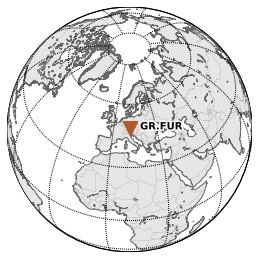In :
from obspy.signal import PPSD

tr = st

ppsd.plot()

/Users/lion/miniconda3/envs/seismo_live/lib/python3.7/site-packages/obspy/signal/spectral_estimation.py:1830: MatplotlibDeprecationWarning:
The set_clim function was deprecated in Matplotlib 3.1 and will be removed in 3.3. Use ScalarMappable.set_clim instead.
cb.set_clim(*fig.ppsd.color_limits)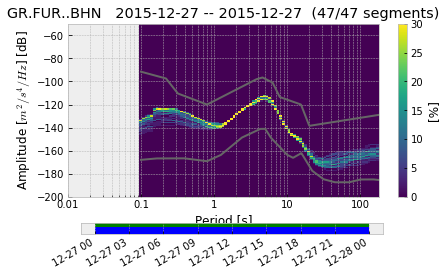Since longer term stacks would need too much waveform data and take way too long to compute, we prepared one year continuous data preprocessed for a single channel of station FUR to play with..

• load long term pre-computed PPSD from file PPSD_FUR_HHN.npz using PPSD's load_npz() staticmethod (i.e. it is called directly from the class, not an instance object of the class)
• plot the PPSD (default is full time-range, depending on how much data and spread is in the data, adjust max_percentage option of plot() option) (might take a couple of minutes..!)
• do a cumulative plot (which is good to judge non-exceedance percentage dB thresholds)
In :
from obspy.signal import PPSD


In :
ppsd.plot(max_percentage=10)
ppsd.plot(cumulative=True)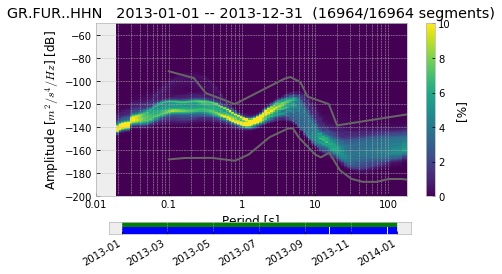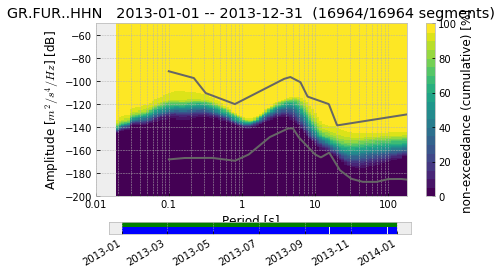• do different stacks of the data using the calculate_histogram() (see docs!) method of PPSD and visualize them
• compare differences in different frequency bands qualitatively (anthropogenic vs. "natural" noise)..
• nighttime stack, daytime stack
• advanced exercise: Use the callback option and use some crazy custom callback function in calculate_histogram(), e.g. stack together all data from birthdays in your family.. or all German holidays + Sundays in the time span.. or from dates of some bands' concerts on a tour.. etc.
In :
ppsd.calculate_histogram(time_of_weekday=[(-1, 0, 2), (-1, 22, 24)])
ppsd.plot(max_percentage=10)
ppsd.calculate_histogram(time_of_weekday=[(-1, 8, 16)])
ppsd.plot(max_percentage=10)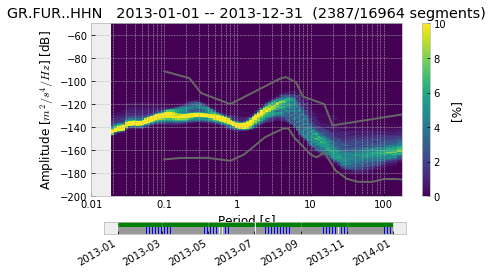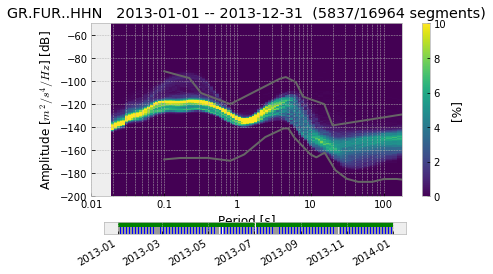• do different stacks of the data using the calculate_histogram() (see docs!) method of PPSD and visualize them
• compare differences in different frequency bands qualitatively (anthropogenic vs. "natural" noise)..
• weekdays stack, weekend stack
In :
ppsd.calculate_histogram(time_of_weekday=[(1, 0, 24), (2, 0, 24), (3, 0, 24), (4, 0, 24), (5, 0, 24)])
ppsd.plot(max_percentage=10)
ppsd.calculate_histogram(time_of_weekday=[(6, 0, 24), (7, 0, 24)])
ppsd.plot(max_percentage=10)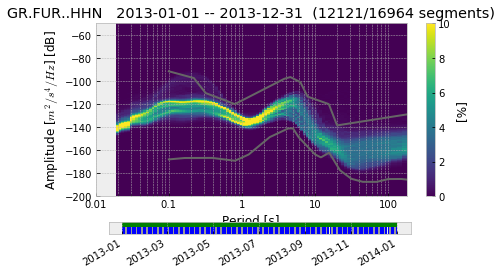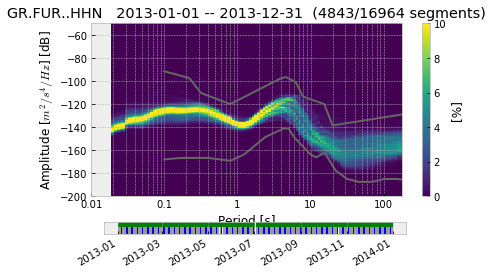• do different stacks of the data using the calculate_histogram() (see docs!) method of PPSD and visualize them
• compare differences in different frequency bands qualitatively (anthropogenic vs. "natural" noise)..
• seasonal stacks (e.g. northern hemisphere autumn vs. spring/summer, ...)
In :
ppsd.calculate_histogram(month=[10, 11, 12, 1])
ppsd.plot(max_percentage=10)
ppsd.calculate_histogram(month=[4, 5, 6, 7])
ppsd.plot(max_percentage=10)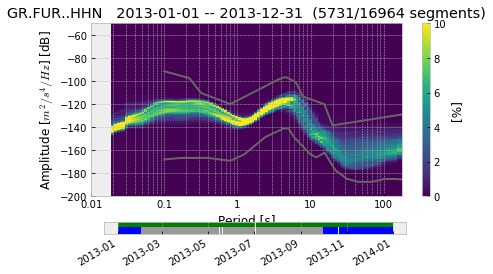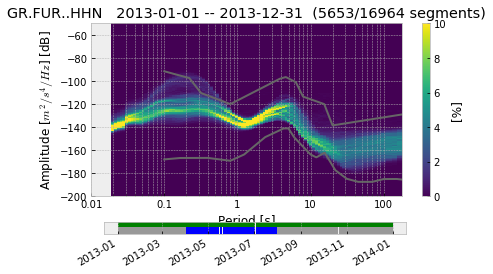• do different stacks of the data using the calculate_histogram() (see docs!) method of PPSD and visualize them
• compare differences in different frequency bands qualitatively (anthropogenic vs. "natural" noise)..
• stacks by specific month
• maybe even combine several of above restrictions.. (e.g. only nighttime on weekends)
In [ ]: